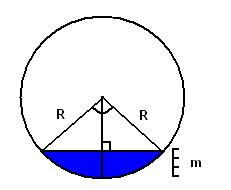# Cylindrical tank volume

Cylindrical tank volume

This is simple calculator which computes volume of liquid in cylindrical tank laying on side. For more advanced version, which computes volume of liquid in tilted tank go to Tilted cylindrical tank volume#### Cylindrical tank volume

Digits after the decimal point: 2
Liquid volume

Percents of total

Total volumeOk, the solution is simple if you look at the picture. (If there are more than half of cylinder filled with liquid, we can compute air volume and subtract from total.)

Total volume of cylinder is

So we need to find area of circle filled with liquid and multiply by height.
Filled area is sector area minus triangle area.

Sector area is
, where alpha is arc angle.

Arc angle is unknown yet. To find it, we draw vertical line from center of circle. It divides triangle to two right-angle triangles with hypotenuse equals to R and top cathet R-m.
Thus,

and

The top triangle is isosceles. The sides equal to R. Let's find its base. The base is two times cathet from right-angle triangle, which is

according to pythagorean theorem.

Now, knowing all triangle sides we can compute its area using, for example, Heron's formula calculator

where

Now we know sector area and triangle area. If we subtract triangle area from sector area we get filled area, multiply it by height and get the answer.

URL copied to clipboard
PLANETCALC, Cylindrical tank volume